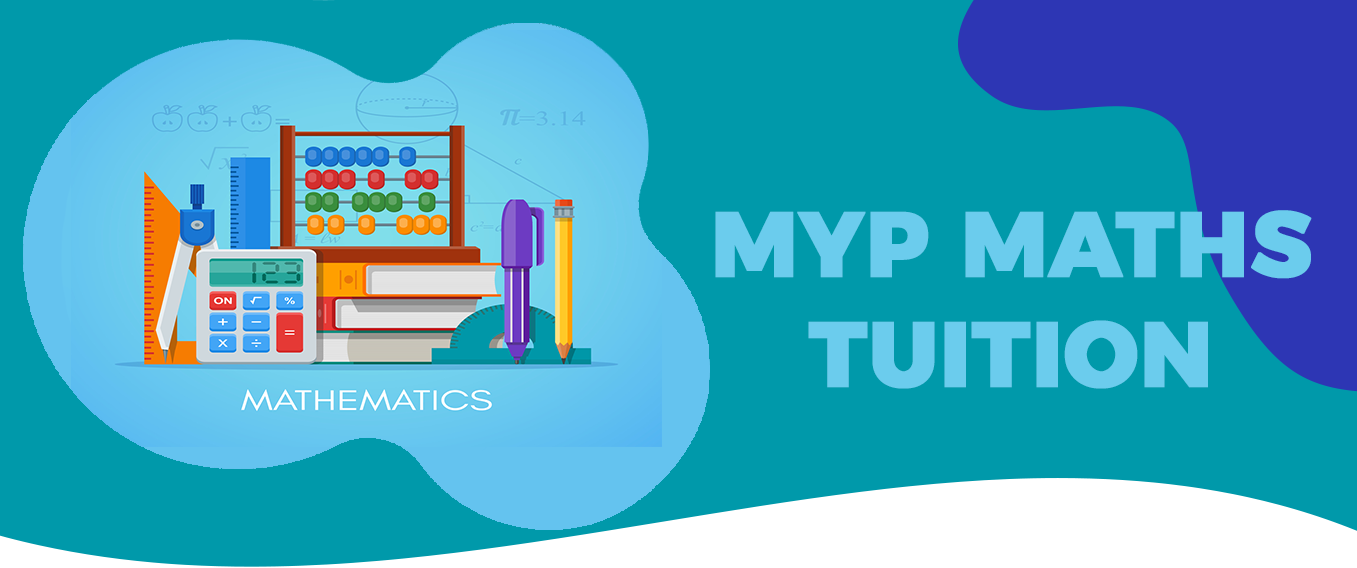# MYP Maths TuitionBaccalaureate Classes provides well qualified and experienced IB MYP maths online tutor across the globe. The IB MYP mathematics is considered as the most important subject of the curriculum. It has two variants which are MYP standard maths and MYP extended maths. The main topics of the course are number, algebra, geometry, trigonometry, statistics and probability. There are many subtopics for every topic. The main aim of the course is to develop a curiosity and understanding towards the basic principles and nature of mathematics so that students can use their skills to solve real world problems or pursue a higher course in mathematics. In this course students are encouraged to provide their critical thoughts and reflections on their work as well as other’s work. Students feel the need of IB MYP maths tuition to understand the use of basic concepts to solve real life situation based problems. The IB MYP extended mathematics has few extra topics and the depth of the topics is more as compared to standard mathematics. The tutoring duration for each MYP subject is 50 hours.

The MYP mathematics on screen examination is based on four assessment criterion which are known as criteria A B C and D. Each criteria has a grading range of 1 to 8. Our IB MYP maths tutor trains the students to develop their problem solving skills as per the defined criterion. Criteria A is called knowing and understand which checks the student’s basic conceptual understanding of various topics. Criteria B of the MYP maths paper is known as investigating patterns. Criteria C of MYP mathematics is based on communicating and it checks the mathematical language and representation of the students. Criteria D is based on application of theoretical knowledge of mathematics in real life contexts. As per this criteria students are supposed to develop problem solving skills and strategies for various types of real world problems and draw logical conclusions reflecting their results. Our team of online MYP maths tutor has developed revision notes and model papers for the students. Our tutors encourage the students to solve many worksheets for each topic to strengthen their question solving skills.

The on screen examination of IB MYP mathematics consists of three tasks. We train the students for the three tasks through our IB MYP maths online tuition and help them to crack the paper pattern. The first task is called knowing and understanding and it is based on criteria A and C. The second task is investigating patterns and it checks criteria B and C. The third task is application of mathematics to solve real life problems and it is based on criteria C and D. The maximum marks for each task is 40 and hence the total marks is 120. Our IB MYP maths online tutor conducts the topic wise e assessment after completion of every topic. Our MYP online maths tutors suggest that the students must be familiar with a variety of questions of based on all four criterions. We provide IB MYP maths home tutor for our local students in Gurgaon and Delhi.